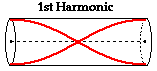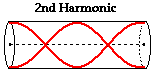# Question #4d966

Apr 17, 2014

In an open-air tube, there is an antinode located at each end, with at least one node between.

According to www.physicsclassroom.com, "the basis for drawing the standing wave patterns for air columns is that vibrational antinodes will be present at any open end and vibrational nodes will be present at any closed end. "

The fundamental frequency of the open-air column has the longest wavelength possible, meaning that the length of the pipe, l, is equal to 1/2 of the wavelength ($l = \frac{1}{2} \lambda$). (The distance between two antinodes in a standing wave is 1/2 $\lambda$.)If the wavelength is halved, it allows a standing wave with three antinodes and two nodes to form in the length of the open air column. This is the second harmonic. The frequency of this sound would be double the first one. In terms of music, this means one octave higher.